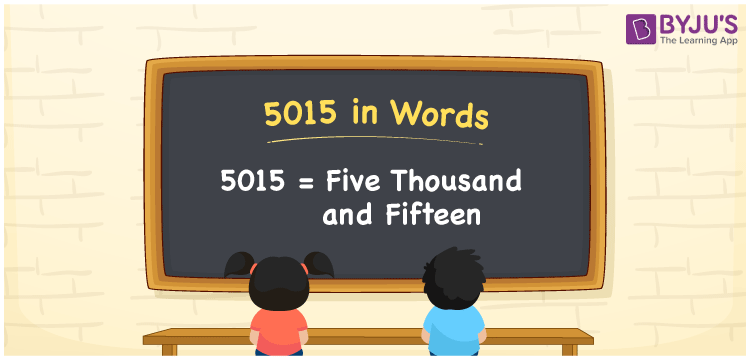# 5015 in Words

5015 in words is written as Five thousand fifteen. In both the International System of Numerals and the Indian System of Numerals, 5015 is written as Five thousand fifteen. The number 5015 is a Cardinal Number as it represents some quantity.

 5015 in Words Five thousand fifteen Five thousand fifteen in Number 5015

## 5015 in English Words

5015 in English words is read as “Five thousand fifteen”.## How to Write 5015 in Words?

To write 5015 in words, we shall use the place value chart. In the place value chart, put 5 in the thousands, 0 in the hundreds, 1 in the tens, and 5 in the ones, respectively. Let us make a place value chart to write the number 5015 in words.

 Thousands Hundreds Tens Ones 5 0 1 5

Thus, we can write the expanded form as

5 × Thousand + 0 × Hundred + 1 × Ten + 5 × One

= 5 × 1000 + 0 × 100 + 1 × 10 + 5 × 1

= 5000 + 0 + 10 + 5

= 5015

= Five thousand fifteen.

5015 is a natural number which is the successor of 5014 and the predecessor of 5016.

5015 in words – Five thousand fifteen

• Is 5015 an odd number? – Yes
• Is 5015 an even number? – No
• Is 5015 a perfect square number? – No
• Is 5015 a perfect cube number? – No
• Is 5015 a prime number? – No
• Is 5015 a composite number? – Yes

## Frequently Asked Questions on 5015 in Words

Q1

### How to write 5015 in words?

5015 in words is written as Five thousand fifteen.
Q2

### How to write 5015 in the International and Indian System of Numerals?

In both, the system of numerals, 5015 in words, is written as Five thousand fifteen.
Q3

### How to write 5015 in a place value chart?

In the place value chart, write 5 in the thousands, 0 in the hundreds, 1 in the tens, and 5 in the ones, respectively.